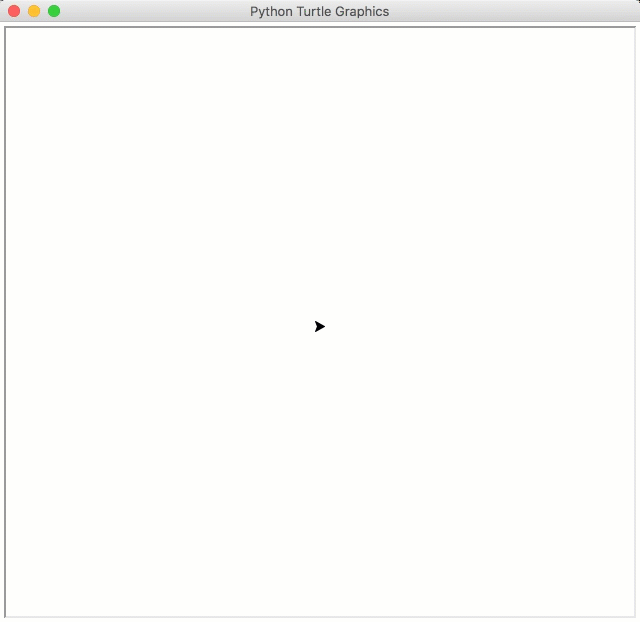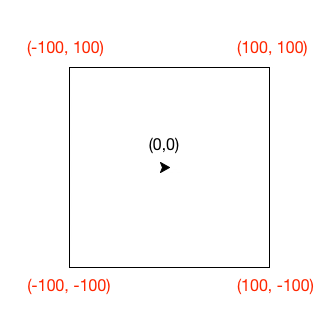# 11. Logical Operators¶

Quick Overview of Day

Introduce the logical operators `and`, `or`, `not`. Work on practice problems, in which students create programs that utilize logical operators. Booleans practice quiz.

## 11.1. `and`, `or`, `not`¶

There are three logical operators: `and`, `or`, and `not`. The semantics (meaning) of these operators is similar to their meaning in English. For example, `x > 0 and x < 10` is true only if `x` is greater than 0 and at the same time, x is less than 10. How would you describe this in words? You would say that x is between 0 and 10, not including the endpoints.

`n % 2 == 0 or n % 3 == 0` is true if either of the conditions is true, that is, if the number is divisible by 2 or divisible by 3. In this case, one, or the other, or both of the parts has to be true for the result to be true.

Finally, the `not` operator negates a boolean expression, so `not  x > y` is true if `x > y` is false, that is, if `x` is less than or equal to `y`.

Caution

Common Mistake!

There is a very common mistake that occurs when programmers try to write boolean expressions. For example, what if we have a variable `number` and we want to check to see if its value is 5,6, or 7. In words we might say: “number equal to 5 or 6 or 7”. However, if we translate this into Python, `number == 5 or 6 or 7`, it will not be correct. The `or` operator must join the results of three equality checks. The correct way to write this is `number == 5 or number == 6 or number == 7`. This may seem like a lot of typing but it is absolutely necessary. You cannot take a shortcut.

## 11.2. Precedence of Operators¶

We have now added a number of additional operators to those we have learned. It is important to understand how these operators relate to the others with respect to operator precedence. Python will always evaluate the arithmetic operators first (** is highest, then multiplication/division, then addition/subtraction). Next comes the relational operators. Finally, the logical operators are done last. This means that the expression `x*5 >= 10 and y-6 <= 20` will be evaluated so as to first perform the arithmetic and then check the relationships. The `and` will be done last. Although many programmers might place parenthesis around the two relational expressions, it is not necessary.

The following table summarizes the precedence discussed so far from highest to lowest.

Level

Category

Operators

7(high)

exponent

**

6

multiplication

*, /, //, %

5

+, -

4

relational

==, !=, <=, >=, >, <

3

logical

not

2

logical

and

1(low)

logical

or

Here is an animation for the above expression:

5 * 3 > 10 and 4 + 6 == 11

## 11.3. Practice Problems¶

### 11.3.1. Under 100¶

Write a program that takes in a number from the user, and correctly prints either “That number is between 1 to 100” or “That number is not between 1 to 100”. You may only use a single if/else block to solve this problem.

Write a program that asks the user which province they live in. If the province isn’t “saskatchewan”, print out “You should come visit Saskatchewan sometime!”. You must use at least one logical operator in your solution.

### 11.3.3. Divisible by 3 or 5¶

Write a program that asks the user to enter a number. You should print out a message to the user, either “That number is divisible by either 3 or 5”, or “That number is not divisible by either 3 or 5”. Be sure to consider the data type of the input you are taking in from the user. Use a single if/else block to solve this problem.

### 11.3.4. Turtle Stuck In a Square¶

You have been given some starter code for the problem below. You only need to change one line of the code, specifically the line containing the while loop. Replace the word `something` with the appropriate boolean questions that will cause the turtle to continue moving until it gets to one of the edges of the square. Each time the program is run, the turtle will pick a new random direction to move. When executed, your program should look something like this:Note that you can get the current x coordinate of the turtle by calling `bob.xcor()`, and the current y coordinate by calling `bob.ycor()`. Also remember that the turtle begins at the origin (0, 0), which is in the center of the screen. The vertices of the square have x and y coordinates as shown below:## 11.4. Booleans Practice Quiz¶

To confirm that you understand the boolean variables, you should try the Booleans Practice Quiz using only your brain (in other words, try to figure out what will happen without running the code).

Next Section - 12. The Time Module and Using a Micro:bit